# Carathéodory-Schur extension problem

(diff) ← Older revision | Latest revision (diff) | Newer revision → (diff)

Letbe a given polynomial. The Carathéodory–Schur extension problem is to find (if possible) an analytic functionon the open unit discsuch thathas a Taylor expansion (cf. Taylor series) of the form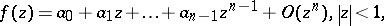(a1)

andis uniformly bounded by one on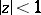. In other words,is an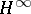-function withof which the firstTaylor coefficients are prescribed. The problem is solvable if and only if the lower-triangular Toeplitz matrixhas operator norm at most one. If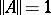, the solution is unique. If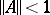, then the number of solutions is infinite, and the set of solutions can be parametrized by a fractional-linear mapping. The necessary and sufficient conditions for the existence of a solution can also be expressed in terms of the so-called Schur numbers, which can be constructed step-by-step from the given coefficients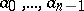(cf. also Carathéodory interpolation). This latter approach, invented originally by I. Schur [a6], [a7], in the beginning of the 19th century, includes recursive methods to obtain the set of all solutions. These recursive methods play an important role in modern operator theory, electrical engineering and geophysics (see [a3], [a4], [a5]). A detailed account of the Carathéodory–Schur problem and its solution may be found in [a3], Chapt. 1.

By multiplying both sides of (a1) by, one sees that the Carathéodory–Schur extension problem for the polynomial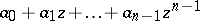is equivalent to the Nehari extension problem for the sequence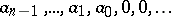. The problem may also be seen as a Nevanlinna–Pick-type interpolation problem, where one seeksof-norm at most one such that the function and its derivatives up to order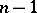have prescribed values at zero (cf. also Nevanlinna–Pick interpolation). The Carathéodory–Schur extension problem has natural generalizations for matrix-valued and operator-valued polynomials (see [a2]). The problem has also a non-stationary variant, in which the role of the polynomial is taken over by a lower-triangular band matrix which has to be completed to a full lower-triangular matrix of operator norm at most one. For this non-stationary variant there is a natural analogue of the Schur numbers, namely the choice sequences, which determine the set of solutions (cf. [a1]).

How to Cite This Entry:
Carathéodory-Schur extension problem. Encyclopedia of Mathematics. URL: http://encyclopediaofmath.org/index.php?title=Carath%C3%A9odory-Schur_extension_problem&oldid=23224
This article was adapted from an original article by I. GohbergM.A. Kaashoek (originator), which appeared in Encyclopedia of Mathematics - ISBN 1402006098. See original article